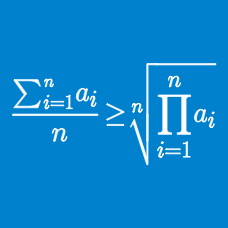Algebra

# Classical Inequalities: Level 4 Challenges

For positive real numbers $a,b,c,d,$ find the minimum value of the following expression:

$\displaystyle (a+b+c+d)\Bigl( \dfrac{25}{a} + \dfrac{36}{b} +\dfrac{81}{c} +\dfrac{144}{d} \Bigr).$

Let $a,b,c,$ and $d$ be real numbers. Find the maximum possible value of $\min\{a-b^2,\ b-c^2,\ c-d^2,\ d-a^2\}.$

Consider all positive reals $a$ and $b$ such that

$ab (a+b) = 2000.$

What is the minimum value of

$\frac{1}{a} + \frac{1}{b} + \frac{1}{a+b} ?$

$a, b$ and $c$ are positive real numbers satisfying $a + b + c = 100$. The maximum value of

$a + \sqrt{ab} + \sqrt{abc}$

can be expressed as $\dfrac{m}{n}$, where $m$ and $n$ are coprime positive integers. What is the value of $m+n$?

$x_1, x_2, x_3, x_4$ and $x_5$ are reals such that $x_1+x_2+x_3+x_4+x_5=8$ and $x_1^2+x_2^2+x_3^2+x_4^2+x_5^2=16.$ What is the largest possible value of $x_5?$

×